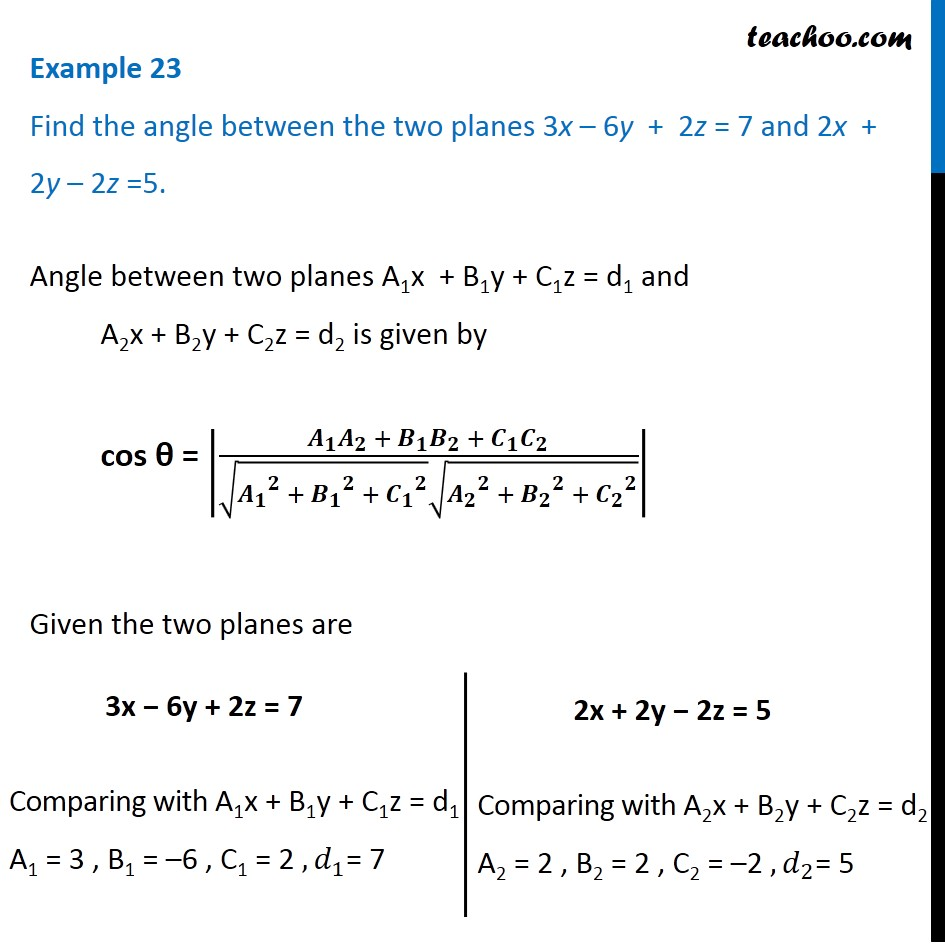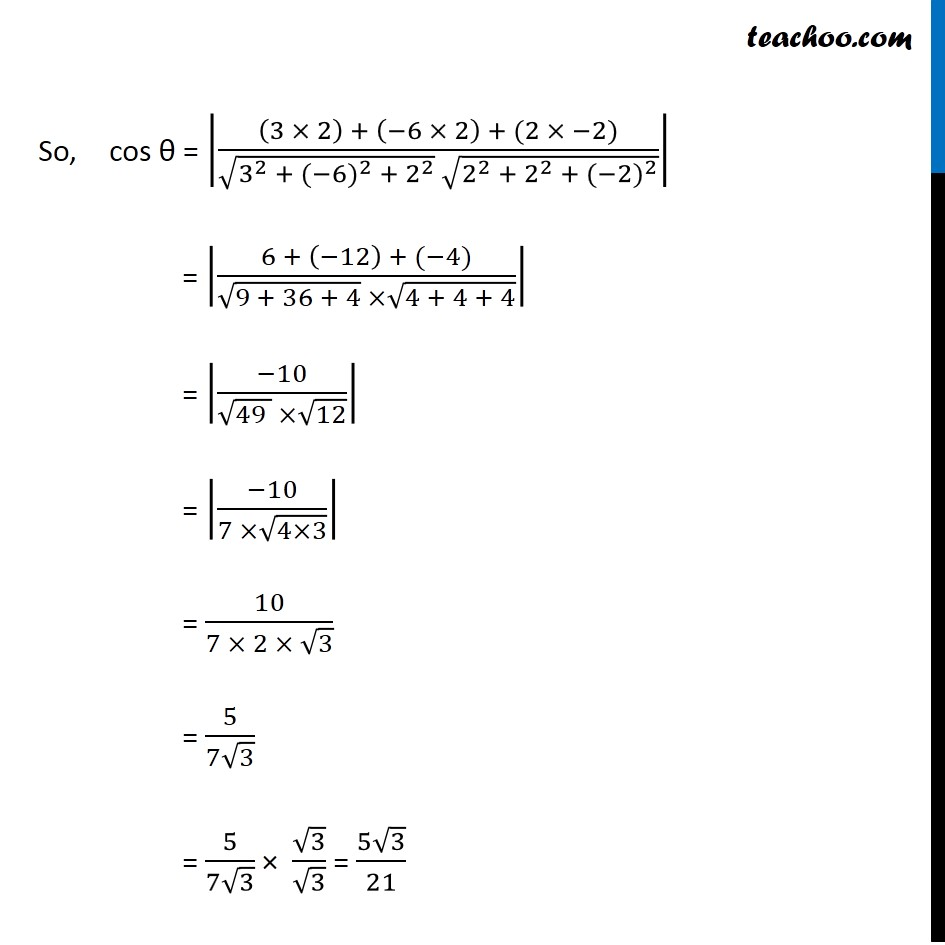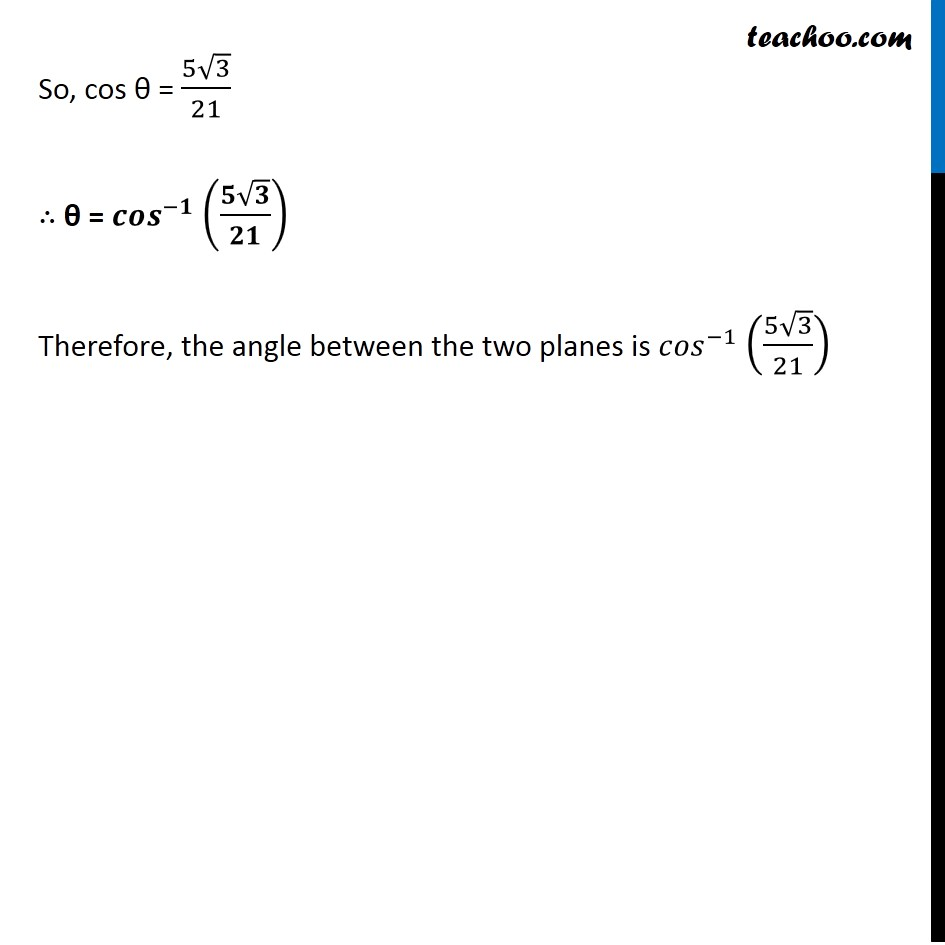Examples

Chapter 11 Class 12 Three Dimensional Geometry
Serial order wiseLearn in your speed, with individual attention - Teachoo Maths 1-on-1 Class

### Transcript

Question 13 Find the angle between the two planes 3x – 6y + 2z = 7 and 2x + 2y – 2z =5.Angle between two planes A1x + B1y + C1z = d1 and A2x + B2y + C2z = d2 is given by cos θ = |(𝑨_𝟏 𝑨_𝟐 + 𝑩_𝟏 𝑩_𝟐 + 𝑪_𝟏 𝑪_𝟐)/(√(〖𝑨_𝟏〗^𝟐 + 〖𝑩_𝟏〗^𝟐 + 〖𝑪_𝟏〗^𝟐 ) √(〖𝑨_𝟐〗^𝟐 + 〖𝑩_𝟐〗^𝟐 + 〖𝑪_𝟐〗^𝟐 ))| Given the two planes are 3x − 6y + 2z = 7 Comparing with A1x + B1y + C1z = d1 A1 = 3 , B1 = –6 , C1 = 2 , 𝑑_1= 7 2x + 2y − 2z = 5 Comparing with A2x + B2y + C2z = d2 A2 = 2 , B2 = 2 , C2 = –2 , 𝑑_2= 5 So, cos θ = |((3 × 2) + (−6 × 2) + (2 × −2))/(√(3^2 + 〖(−6)〗^2 + 2^2 ) √(2^2 + 2^2 + 〖(−2)〗^2 ))| = |(6 + (−12) + (−4))/(√(9 + 36 + 4) ×√(4 + 4 + 4))| = |(−10)/(√(49 ) ×√12)| = |(−10)/(7 ×√(4×3))| = 10/(7 × 2 × √3) = 5/(7√3) = 5/(7√3) × √3/√3 = (5√3)/21 So, cos θ = (5√3)/21 ∴ θ = 〖𝒄𝒐𝒔〗^(−𝟏) ((𝟓√𝟑)/𝟐𝟏) Therefore, the angle between the two planes is 〖𝑐𝑜𝑠〗^(−1) ((5√3)/21) E